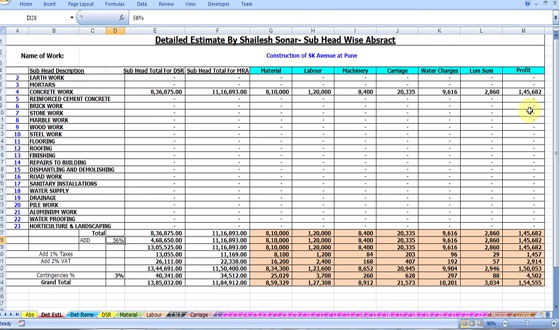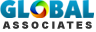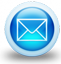# Cost Control Technic for Construction Projects

If you want to get the answer of those questions, then this is the must watch video for you.

• How to quote Item Rate Tender?
• How to calculate its Market Rate Analysis?
• How to calculate resource Cost Breakup?
• This program is very useful for calculating Earn Value Analysis, Cost Control & Resource Management.
• This program contains more than 20 million links & calculations which are interconnected, more than 10 thousand formulae which are used, total 4000 thousand Civil item rate analysis based on standard rate analysis. It includes all type of civil Engineering Calculations.# Estimating Sheets